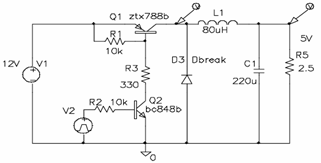# DC-DC Step Down Converter Circuit Diagram using ZTX788B

DC-DC Step Down Converter Circuit Diagram using ZTX788B -The following schematic diagrams DC-DC Step Down Converter using ZTX788B PNP silicon planar tDC-DC Step Down Converter Circuit Diagram using ZTX788B -The following schematic diagrams DC-DC Step Down Converter using ZTX788B PNP silicon planar transistor as a switch element in a 12V to 5V circuit.

The circuit shows the general form of the step down or ‘Buck’ converter using a PNP transistor operating at 100kHz and supplies a 2A load.

Complete read about DC-DC Step Down Converter Circuit Diagram using ZTX788B can be download here or you might need the ZTX788B datasheet (source: diodes.com)

Tags: silicon planar transistor, ZTX788B datasheet, DC DC step down converter,

Copyright www.unboxing.eu.org